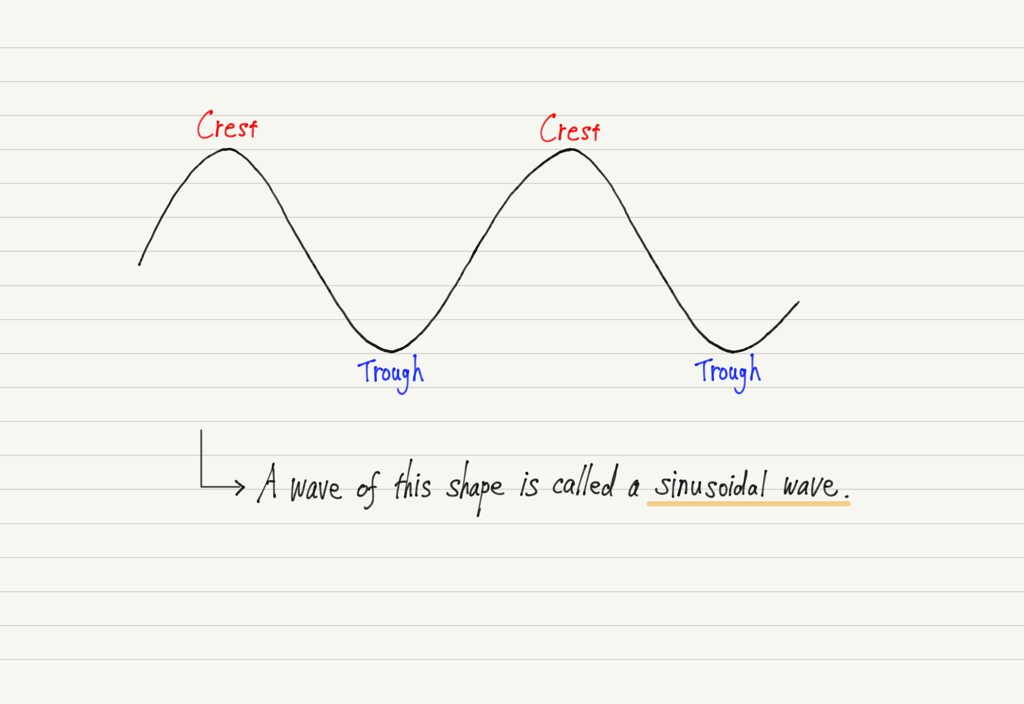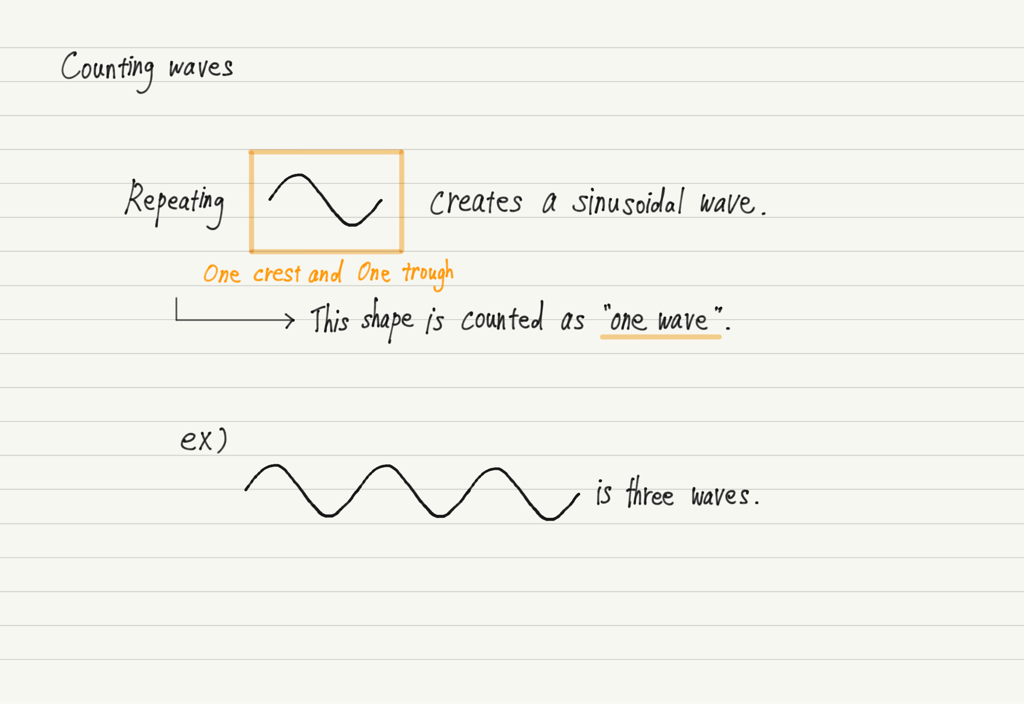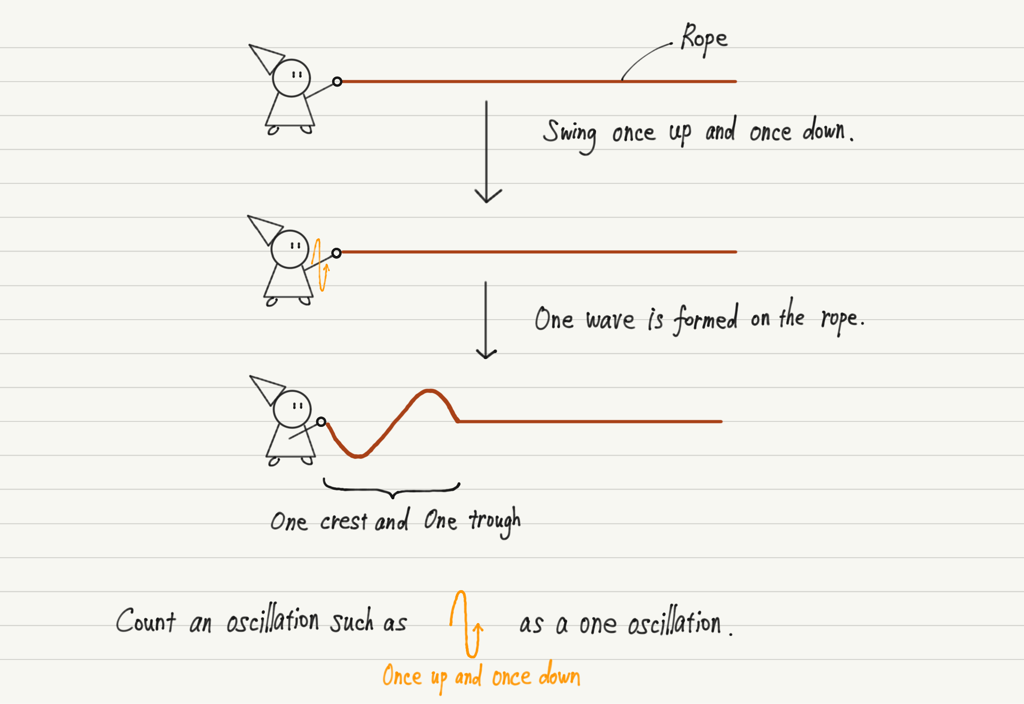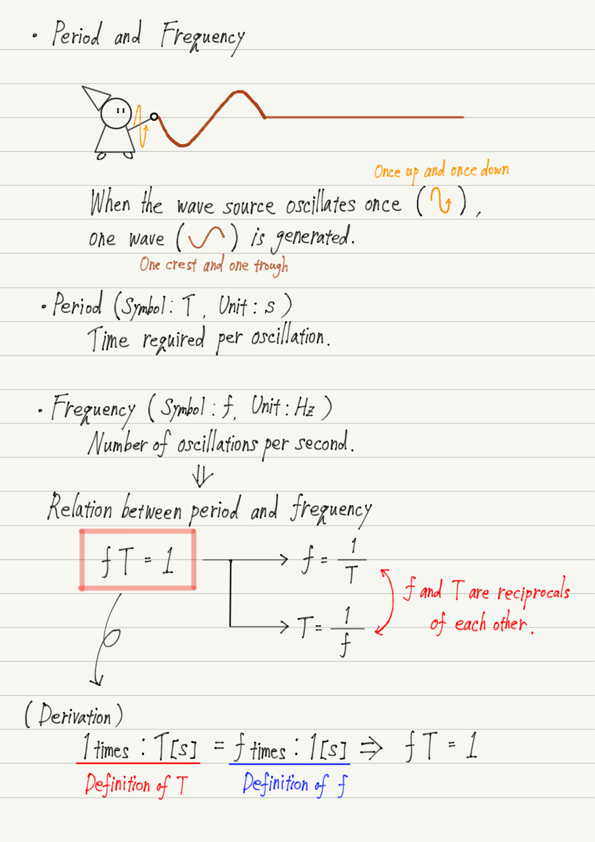# Period and Frequency

In this lecture, we will introduce some frequently used terms in the field of waves.

## Sinusoidal wave

In high school physics, we mostly deal with the simplest wave, called sinusoidal wave.As you can see in the figure above, the sinusoidal wave has alternating crest and trough and repeats the same shape.
We will count the number of waves according to how many times this “set of crests and troughs” is repeated.Let’s think specifically in terms of waves traveling down a rope.

Holding the end of the rope by hand and swinging it up and down produces a sinusoidal wave in the rope.
As the rope continues to swing, waves are created one after another.
Waves created in this way are called continuous wave.
On the other hand, those that stop swinging halfway through are called pulse wave.

We will also count the swing of the arm that creates one wave on the rope as one oscillation.## Period

The time it takes to vibrate a medium once is called the period.

For example,
Slow swing of the rope → Long period,
Quick swing of the rope → Short period.

## Frequency

The number of times a medium vibrates per second is called the frequency.

For example,
Slow swing of the rope → Low frequency,
Quick swing of the rope → High frequency.

## Relation between period and frequency

Let’s reiterate what I wrote above.

Slow swing of the rope → Long period/Low frequency,
Quick swing of the rope → Short period/High frequency.

There seems to be some relationship between period and frequency.
So, let’s check the definition again.

Period is T[s] → The medium oscillates once every T seconds.

Frequency is f[Hz] → The medium vibrates f times per second.

Since the key points in both cases are the number of oscillations and the time of oscillation, let’s create a ratio of the number of oscillations to the time.

Period is T[s] → Number of oscillations: time = 1 : T ,

Frequency is f[Hz] → Number of oscillations: time = f : 1 .

Since both of these ratios represent the same ratio,

1 ： Tf ： 1

From this equation, we see that the relationship fT = 1 holds between the period T and the frequency f.
This relation is used frequently, so please memorize it!

## Summary of this lecture## Next Time

In the next lecture, we will do calculations related to waves.

https://www.yukimura-physics.com/entry/wave-f03

error: Content is protected !!